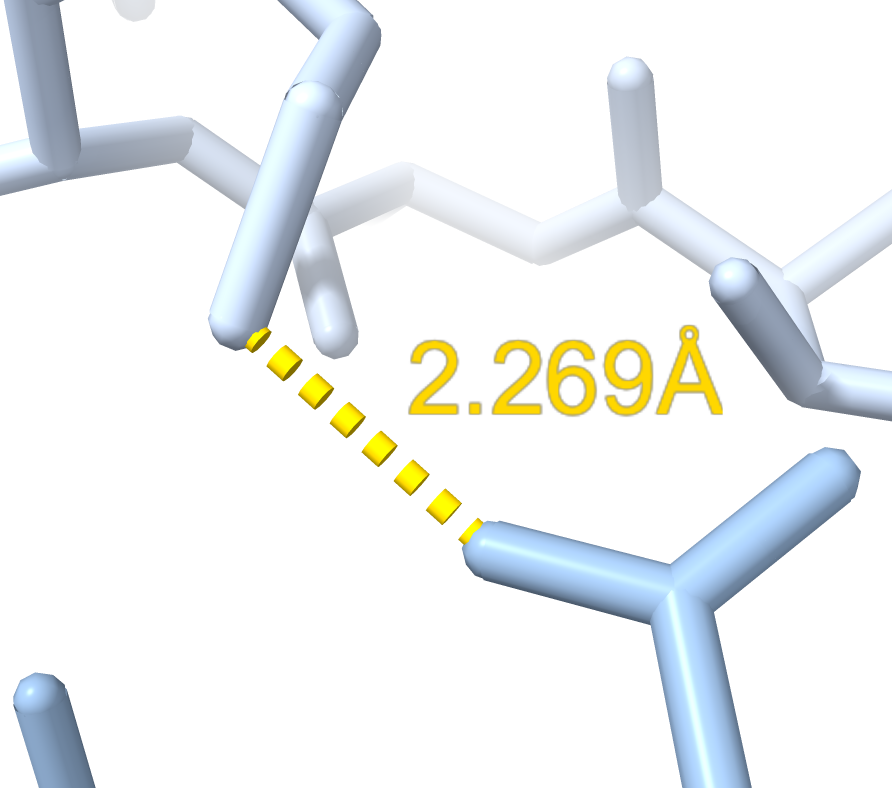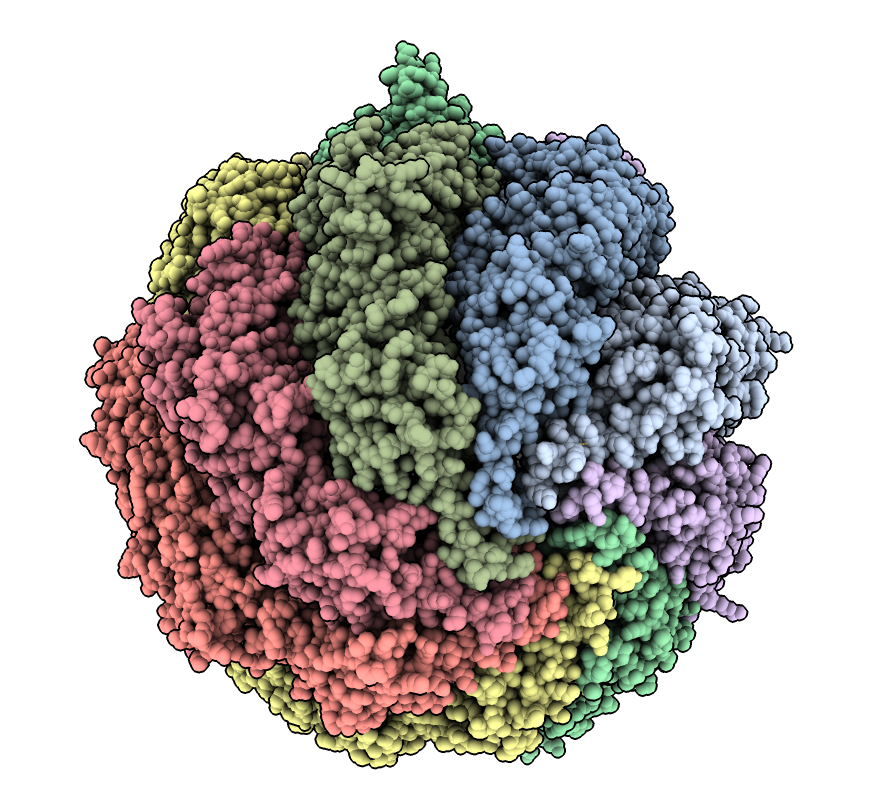# Find closest pair of atoms

Here is Python code defining a command “closest” that finds the closest pair of atoms between two set of atoms. Opening the closest.py code in ChimeraX adds the command

``````open closest.py
``````

then use the command on two sets of atoms

``````open 7LUP
closest /A to /B show true

Minimum distance 2.27 between /A LYS 45 NZ and /B ASP 522 OD1
``````Here is the closest.py code:

``````#
# Add command "closest" that finds the closest pair of atoms given two
# sets of atoms.
#
# open 7LUP
# closest /A to /B
#
#  Minimum distance 2.27 between /A LYS 45 NZ and /B ASP 522 OD1
#

def closest(session, atoms, to_atoms, max_dist = 10, show = False):
xyz1, xyz2 = atoms.scene_coords, to_atoms.scene_coords
from chimerax.geometry import find_closest_points
i1, i2, nearest1 = find_closest_points(xyz1, xyz2, max_dist)
if len(nearest1) == 0:
d = a1 = a2 = None
else:
v = xyz2[nearest1] - xyz1[i1]
d2 = (v*v).sum(axis=1)
c = d2.argmin()
c1, c2 = i1[c], nearest1[c]
a1, a2 = atoms[c1], to_atoms[c2]
from math import sqrt
d = sqrt(d2[c])
report_closest(session, d, a1, a2, show, max_dist)
return d, a1, a2

def report_closest(session, d, a1, a2, show, max_dist):
if d is None:
msg = 'No atom pairs within distance %.3g of each other' % max_dist
else:
msg = 'Minimum distance %.2f between %s and %s' % (d, str(a1), str(a2))
session.logger.status(msg, log = True)

if show and a1 and a2:
from chimerax.core.commands import run
run(session, 'distance %s %s' % (a1.atomspec, a2.atomspec))
run(session, 'view %s %s' % (a1.atomspec, a2.atomspec))

def closest_slow(session, atoms, to_atoms, max_dist = 10, show = False):
'''Loop through every pair of atoms in Python.  This is slow.'''
dmin = amin1 = amin2 = None
from chimerax.geometry import distance
for a1 in atoms:
for a2 in to_atoms:
d = distance(a1.scene_coord, a2.scene_coord)
if (dmin is None or d < dmin) and d <= max_dist:
dmin, amin1, amin2 = d, a1, a2
report_closest(session, dmin, amin1, amin2, show, max_dist)
return dmin, amin1, amin2

def register_command(session):
from chimerax.core.commands import CmdDesc, register, FloatArg, BoolArg
from chimerax.atomic import AtomsArg
desc = CmdDesc(required= [('atoms', AtomsArg)],
keyword = [('to_atoms', AtomsArg),
('max_dist', FloatArg),
('show', BoolArg)],
required_arguments = ['to_atoms'],
synopsis = 'find closest pair of atoms')
register('closest', desc, closest, logger=session.logger)

register_command(session)
``````

Tom Goddard, Sptember 28, 2021